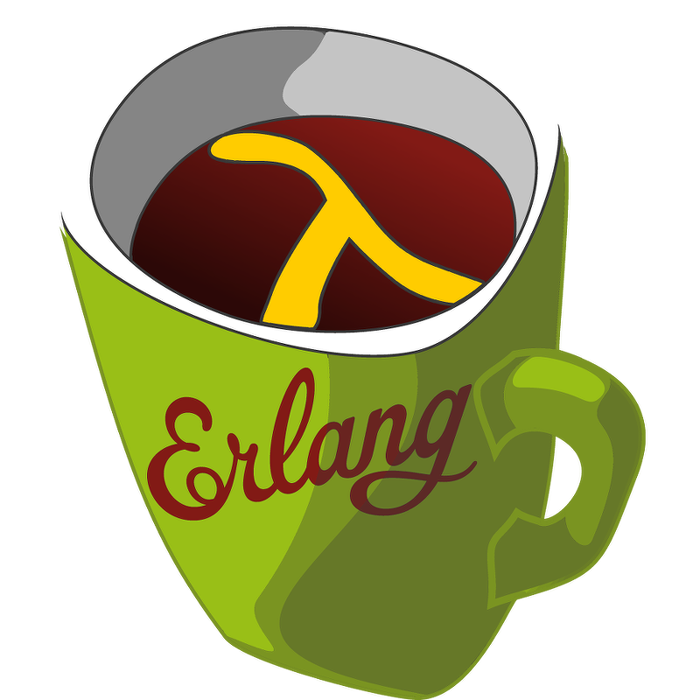This week's LFE Friday was translated with permission from the Erlang Thursday series by Steven Proctor. This week's translator: Robert Virding.

Today's LFE Friday from a Sweden celebrating midsummer is on queue:split/2 from the `queue` modules Original API.

`queue:split/2` takes two arguments. The first argument being a integer n from 0 to size, where size is number of items in the queue, and the second argument is the queue that we wish to split. The return value is a two-tuple with the first element being a queue of the first n items, and the second element of the tuple is a queue of the rest of the items.

``````> (set queue-one (queue:from_list '(a 1 b 2 c 3 4)))
#((4 3 c) (a 1 b 2))
> (queue:split 4 queue-one)
#(#((2) (a 1 b)) #((4 3) (c)))
> (queue:split 0 queue-one)
#(#(() ()) #((4 3 c) (a 1 b 2)))
> (queue:split 1 queue-one)
#(#(() (a)) #((4 3 c) (1 b 2)))
> (queue:split 7 queue-one)
#(#((4 3 c) (a 1 b 2)) #(() ()))
> (queue:split 15 queue-one)
in (: queue split 15 #((4 3 c) (a 1 b 2)))

> (set (tuple split-first split-second) (queue:split 3 queue-one))
#(#((b 1) (a)) #((4 3 c) (2)))
> split-first
#((b 1) (a))
> split-second
#((4 3 c) (2))
> (queue:peek split-first)
#(value a)
> (queue:peek split-second)
#(value 2)``````

Erlang also provides a queue:join/2 function that takes two queues, and returns a new queue, with the queue that was passed as the second argument appended to the queue passed in as the first argument.

``````> (queue:join split-first split-second)
#((4 3 c) (a 1 b 2))
> (queue:join split-second split-first)
#((b 1) (2 c 3 4 a))
> (queue:join (queue:new) split-first)
#((b 1) (a))
> (queue:join (queue:new) (queue:new))
#(() ())``````

-Proctor, Robert

20 June 2015

tutorials